Home | | Physics 12th Std | Ohm‚Äôs Law

# Ohm‚Äôs Law

The ohm‚Äôs law can be derived from the equation J = œÉE.

OHM‚ÄôS LAW

The ohm‚Äôs law can be derived from the equation J = œÉE. Consider a segment of wire of length l and cross sectional area A as shown in Figure 2.7.When a potential difference V is applied across the wire, a net electric field is created in the wire which constitutes the current. For simplicity, we assume that the electric field is uniform in the entire length of the wire, the potential difference (voltage V) can be written as

V = El

As we know, the magnitude of current densityBut J = I /A  , so we write the equation (2.14) as

I/A = œÉ V/l .

By rearranging the above equation, we getThe quantity l/œÉA is called resistance of the conductor and it is denoted as R. Note that the resistance is directly proportional to the length of the conductor and inversely proportional to area of cross section.Therefore, the macroscopic form of ohm‚Äôs law can be stated as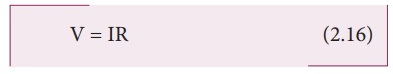From the above equation, the resistance is the ratio of potential difference across the given conductor to the current passing through the conductor.The SI unit of resistance is ohm (Œ©). From the equation (2.16), we infer that the graph between current versus voltage is straight line with a slope equal to the inverse of resistance R of the conductor. It is shown in the Figure 2.8 (a).Materials for which the current against voltage graph is a straight line through the origin, are said to obey Ohm‚Äôs law and their behaviour is said to be ohmic as shown in Figure 2.8(a). Materials or devices that do not follow Ohm‚Äôs law are said to be non-ohmic. These materials have more complex relationships between voltage and current. A plot of I against V for a non-ohmic material is non-linear and they do not have a constant resistance (Figure 2.8(b)).

EXAMPLE 2.5

A potential difference across 24 Œ© resistor is 12 V. What is the current through the resistor?

Solution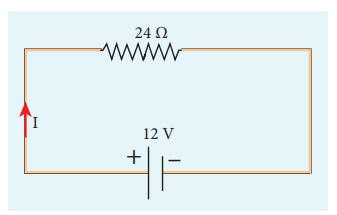V = 12 V and R = 24 Œ©

Current, I = ?

From Ohm‚Äôs law, I = V/R = 12/24 = 0 .5 A

## 1.  Resistivity

In the previous section, we have seen that the resistance R of any conductor is given bywhere œÉ is called the conductivity of the material and it depends only on the type of the material used and not on its dimension.

The resistivity of a material is equal to the reciprocal of its conductivity.Now we can rewrite equation (2.18) using equation (2.19)The resistance of a material is directly proportional to the length of the conductor and inversely proportional to the area of cross section of the conductor. The proportionality constant œÅ is called the resistivity of the material.If l = 1 m and A = 1 m2, then the resistance R = œÅ. In other words, the electrical resistivity of a material is defined as the resistance offered to current flow by a conductor of unit length having unit area of cross section. The SI unit of œÅ is ohm-metre (Œ© m). Based on the resistivity, materials are classified as conductors, insulators and semi-conductors. The conductors have lowest resistivity, insulators have highest resistivity and semiconductors have resistivity greater than conductors but less than insulators. The typical resistivity values of some conductors, insulators and semiconductors are given in the Table 2.1EXAMPLE 2.6

The resistance of a wire is 20 Œ©. What will be new resistance, if it is stretched uniformly 8 times its original length?

Solution

R1 = 20 Œ©, R2= ?

Let the original length (l1) be l.

The new length, l2 = 8l1 (i.,e) l2 =8l

The original resistance, R = œÅ [ l1 / A1]

The new resistance

R2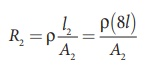Though the wire is stretched, its volume is unchanged.

Initial volume = Final volume

A1l1= A2l2 , A1l = A28l

A1 / A2 = 8l / l = 8

By dividing equation R2 by equation R1, we getSubstituting the value of A1/A2, we get

R2 / R1  = 8 √ó8 = 64 2

R2 = 64 √ó 20=1280 Œ©

Hence, stretching the length of the wire has increased its resistance.

EXAMPLE 2.7

Consider a rectangular block of metal of height A, width B and length C as shown in the figure.If a potential difference of V is applied between the two faces A and B of the block (figure (a)), the current IAB is observed. Find the current that flows if the same potential difference V is applied between the two faces B and C of the block (figure (b)). Give your answers in terms of IAB.

Solution

In the first case, the resistance of the block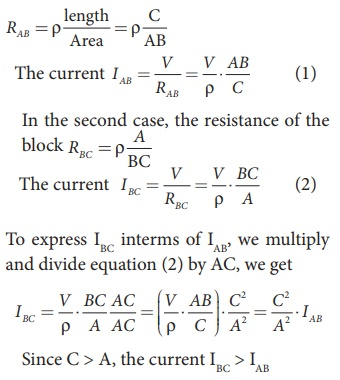2. Resistors in series and parallel

An electric circuit may contain a number of resistors which can be connected in different ways. For each type of circuit, we can calculate the equivalent resistance produced by a group of individual resistors.

### Resistors in series

When two or more resistors are connected end to end, they are said to be in series. The resistors could be simple resistors or bulbs or heating elements or other devices. Figure 2.9 (a) shows three resistors R1, R2 and R3 connected in series.

The amount of charge passing through resistor R1 must also pass through resistors Rand R3 since the charges cannot accumulate anywhere in the circuit.Due to this reason, the current I passing through all the three resistors is the same. According to Ohm‚Äôs law, if same current pass through different resistors of different values, then the potential difference across each resistor must be different. Let V1, V2 and V3 be the potential difference (voltage) across each of the resistors R1, R2 and R3 respectively, then we can write V1 = IR1, V2 = IR2 and V3 = IR3. But the total voltage V is equal to the sum of voltages across each resistor.where RS is the equivalent resistance,

When several resistances are connected in series, the total or equivalent resistance is the sum of the individual resistances as shown in the Figure 2.9 (b).

Note: The value of equivalent resistance in series connection will be greater than each individual resistance.

EXAMPLE 2.8

Calculate the equivalent resistance for the circuit which is connected to 24 V battery and also find the potential difference across 4 Œ© and 6 Œ© resistors in the circuit.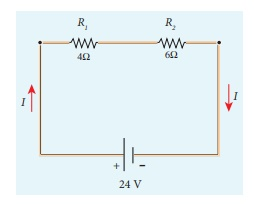Solution

Since the resistors are connected in series, the effective resistance in the circuit

The Current I in the circuit= V/ Req = 24/10 = 2 .4 A

V1 = IR1 = 2 . 4 A√ó 4 Œ© = 9.6V

V2 = IR1 = 2 . 4 A√ó 6 Œ© =14 .4V

### Resistors in parallel

Resistors are in parallel when they are connected across the same potential difference as shown in Figure 2.10 (a).

In this case, the total current I that leaves the battery is split into three separate paths. Let I1, I2 and I3 be the current through the resistors R1, R2 and R3 respectively. Due to the conservation of charge, total current in the circuit I is equal to sum of the currents through each of the three resistors.Since the voltage across each resistor is the same, applying Ohm‚Äôs law to each resistor, we haveSubstituting these values in equation (2.24),we getHere RP is the equivalent resistance of the parallel combination of the resistors. Thus, when a number of resistors are connected in parallel, the sum of the reciprocal of the values of resistance of the individual resistor is equal to the reciprocal of the effective resistance of the combination as shown in the Figure 2.10 (b)Note: The value of equivalent resistance in parallel connection will be lesser than each individual resistance.

House hold appliances are always connected in parallel so that even if one is switched off, the other devices could function properly.

EXAMPLE 2.9

Calculate the equivalent resistance in the following circuit and also find the current I, I1 and I2 in the given circuit.Solution

Since the resistances are connected in parallel, therefore, the equivalent resistance in the circuit isThe resistors are connected in parallel, the potential (voltage) across each resistor is the same.The current I is the total of the currents in the two branches. Then,

I = I1 + I2= 6 A + 4 A = 10 A

EXAMPLE 2.10

When two resistances connected in series and parallel their equivalent resistances are 15 Œ© and 56/15 Œ© respectively. Find the individual resistances.

Solution

Rs = R1 + R2 = 15 Œ© (1)The above equation can be solved using factorisation.

R12-8 R1-7 R1+ 56 = 0

R1 (R1‚Äì 8) ‚Äì 7 (R1‚Äì 8) = 0

(R1‚Äì 8) (R1‚Äì 7) = 0

using in equation (1)

8 + R2 = 15

R2 = 15 ‚Äì 8 = 7 Œ© ,

Substituting in equation (1)

7 + R2 = 15

R2 = 8 Œ© , i.e , (when R1 = 8 Œ© ; R2 = 7 Œ© )

EXAMPLE 2.11

Calculate the equivalent resistance between A and B in the given circuit.SolutionEXAMPLE 2.12

Five resistors are connected in the configuration as shown in the figure. Calculate the equivalent resistance between the points a and b.Solution

Case (a)

To find the equivalent resistance between the points a and b, we assume that current is entering the junction a. Since all the resistances in the outside loop are the same (1Œ©), the current in the branches ac and ad must be equal. So the electric potential at the point c and d is the same hence no current flows into 5 Œ© resistance. It implies that the 5 Œ© has no role in determining the equivalent resistance and it can be removed. So the circuit is simplified as shown in the figure.The equivalent resistance of the circuit between a and b is Req = 1Œ©

## 3. Color code for CarbonresistorsCarbon resistors consists of a ceramic core, on which a thin layer of crystalline carbon is deposited as shown in Figure 2.11. These resistors are inexpensive, stable and compact in size. Color rings are used to indicate the value of the resistance according to the rules given in the Table 2.2.

Three coloured rings are used to indicate the values of a resistor: the first two rings are significant figures of resistances, the third ring indicates the decimal multiplier after them. The fourth color, silver or gold, shows the tolerance of the resistor at 10% or 5% as shown in the Figure 2.12 .If there is no fourth ring, the tolerance is 20%.For the resistor shown in Figure 2.12, the first digit = 5 (green), the second digit = 6 (blue), decimal multiplier = 103 (orange) and tolerance = 5% (gold). The value of resistance = 56 √ó 103 ‚Ñ¶ or 56 k‚Ñ¶ with the tolerance value 5%.## 4. Temperaturedependence of resistivity

The resistivity of a material is dependent on temperature. It is experimentally found that for a wide range of temperatures, the resistivity of a conductor increases with increase in temperature according to the expression,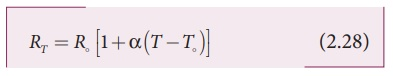where œÅT is the resistivity of a conductor at T oC, œÅo is the resistivity of the conductor at some reference temperature T¬∫ (usually at 20¬∫C) and Œ± is the temperature coefficient of resistivity. It is defined as the ratio of increase in resistivity per degree rise in temperature to its resistivity at T¬∫.

From the equation (2.27), we can writewhere ‚àÜœÅ = œÅT ‚Äì œÅo is change in resistivity for a change in temperature ‚àÜT = T ‚Äì To. Its unit is per oC.

Œ± of conductors

For conductors Œ± is positive. If the temperature of a conductor increases, the average kinetic energy of electrons in the conductor increases. This results in more frequent collisions and hence the resistivity increases. The graph of the equation (2.27) is shown in Figure 2.13

Even though, the resistivity of conductors like metals varies linearly for wide range of temperatures, there also exists a non-linear region at very low temperatures. The resistivity approaches some finite value as the temperature approaches absolute zero as shown in Figure 2.13(b).

As the resistance is directly proportional to resistivity of the material, we can also write the resistance of a conductor at temperature T ¬∫C as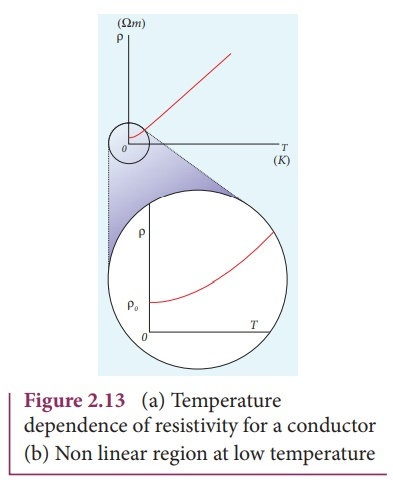The temperature coefficient can be also be obtained from the equation (2.28),where          R = RT ‚àíR is change in resistance during the change in temperature  ŒîT = T ‚ÄìT

Œ± of of semiconductors

For semiconductors, the resistivity decreases with increase in temperature. As the temperature increases, more electrons will be liberated from their atoms (Refer unit 9 for conduction in semi conductors). Hence the current increases and therefore the resistivity decreases as shown in Figure 2.14. A semiconductor with a negative temperature coefficient of resistance is called a thermistor.

The typical values of temperature coefficients of various materials are given in table 2.3.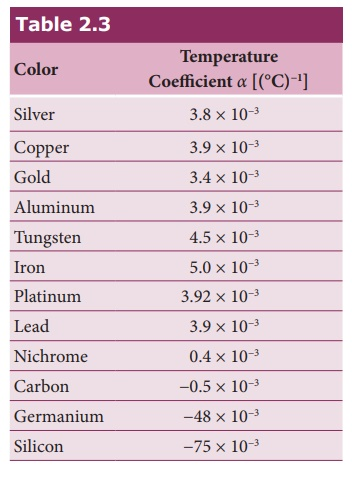We can understand the temperature dependence of resistivity in the following way. In section 2.1.3, we have shown thatthe electrical conductivity, œÉ = ne2œÑ / m . As the m resistivity is inverse of œÉ, it can be written as,The resistivity of materials is

i) inversely proportional to the number density (n) of the electrons

ii) inversely proportional to the average time between the collisions (œÑ).

In metals, if the temperature increases, the average time between the collision (œÑ) decreases and n is independent of temperature. In semiconductors when temperature increases, n increases and œÑ decreases, but increase in n is dominant than decreasing œÑ, so that overall resistivity decreases.EXAMPLE 2.13

If the resistance of coil is 3 Œ© at 20 0C and Œ± = 0.004/0C then determine its resistance at 100 0C.

Solution

R0= 3 Œ©,‚ÄÉ T = 100¬∫C,‚ÄÉ T0 = 20¬∫C

Œ± = 0.004/¬∫C,‚ÄÉ RT = ?

RT= R0(1 + Œ±(T-T0))

R100 = 3(1 + 0.004 √ó 80)

R100 = 3(1 + 0.32)

R100 = 3(1.32)

EXAMPLE 2.14

Resistance of a material at 10¬∫C and 40¬∫C are 45 Œ© and 85 Œ© respectively. Find its temperature co-efficient of resistance.

Solution

T0 = 10¬∫C, T = 40¬∫C, R0= 45 Œ© , R = 85 Œ©

Œ± = 1/R . ŒîR /ŒîTŒ± = 0.0296 per ¬∫C

Tags : Explanation, Formulas, Solved Example Problems , 12th Physics : Current Electricity
Study Material, Lecturing Notes, Assignment, Reference, Wiki description explanation, brief detail
12th Physics : Current Electricity : Ohm‚Äôs Law | Explanation, Formulas, Solved Example Problems